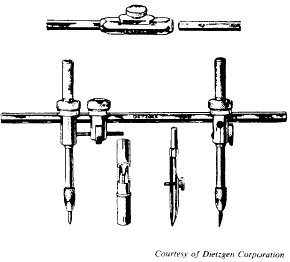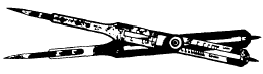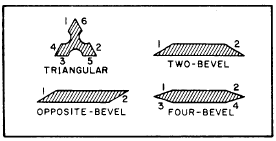Custom SearchMaintenance of Compasses and DividersBasic EngineeringArchitects ScaleBeam Compass

The BEAM COMPASS (fig. 2-21) is used for drawing circles with radii larger than can be set on a pivot joint or bow compass. Both the needle-point attachment and the pen or pencil attachment on a beam compass are slide-mounted on a metalFigure 2-21.-Beam compass.Figure 2-22.-Proportional dividers.

bar called a beam. The slide-mounted attachments can be locked in any desired position on the beam. Thus, a beam compass can be used to draw circles of any radius up to the length of the beam. With one or more beam extensions, the length of the radius of a beam compass ranges from about 18 in. to 70 in.

PROPORTIONAL DIVIDERS

PROPORTIONAL DIVIDERS (fig. 2-22) are used for transferring measurements from one scale to another. This capability is necessary when drawings are to be made to a larger or smaller scale. They can also be used to divide lines or circles into equal parts.

Proportional dividers consist of two legs of equal length, pointed at each end, and held together by a movable pivot. By varying the position of the pivot, you can adjust the lengths of the legs on opposite sides of the pivot so that the ratio between them is equal to the ratio between two scales. Therefore, a distance spanned by the points of one set of legs has the same relation to the distance spanned by the points of the other set as one scale has to the other. On the proportional dividers, a thumb nut moves the pivot in a rack-and-gear arrangement. When the desired setting is reached, a thumb-nut clamp on the opposite side of the instrument locks the pivot in place. A scale and vernier are provided on one leg to facilitate accurate setting. On less expensive models, the movable pivot is not on a rack and gear, and there is no vernier. The dividers may be set by reference to the table of settings that is furnished with each pair; they will accommodate varying ranges of scales from 1:1 to 1:10. However, it is better not to depend entirely on the table of settings. You can check the adjustment by drawing lines representing the desired proportionate lengths, and then applying the points of the instrument to them in turn until, by trial and error, the correct adjustment is reached.

To divide a line into equal parts, set the divider to a ratio of 1 to the number of parts desired on the scale marked Lines. For instance, to divide a line into three parts, set the scale at 3. Measure off the length with points of the longer end. The span of the points at the opposite ends will be equal to one-third the measured length. To use proportional dividers to transfer measurements from feet to meters, draw a line 1 unit long and another line 3.28 units long and set the dividers by trial and error accordingly.

Some proportional dividers have an extra scale for use in getting circular proportions. The scale marked Circle indicates the setting for dividing the circumference into equal parts.

The points of the dividers are of hardened steel, and if they are handled carefully, these points will retain their sharpness during long use. If they are damaged, they may be sharpened and the table of settings will still be usable, but the scale on the instrument will no longer be accurate.

SCALES

In one sense, the term scale means the succession of graduations on any graduated standard of linear measurement, such as the graduations on a steel tape or a thermometer. In another sense, when we refer to the "scale of a drawing," the term means the ratio between the dimensions of the graphic representation of an object and the corresponding dimensions of the object itself.

Suppose, for example, that the top of a rectangular box measures 6 in. by 12 in. If you draw a 6-in. by 12-in. rectangle on the paper, the dimensions of the drawing would be the same as those of the object. The drawing would, therefore, be a full-scale drawing. This scale could be expressed fractionally as 1/1, or it could be given as 1 in. = 1 in.

Suppose that instead of making a full-scale drawing, you decided to make a half-scale drawing. You should then draw a 3-in. by 6-in. rectangle on the paper. This scale could be expressed fractionally as 1/2, or it could be given as 1 in. = 2 in., or as 6 in. = 1 ft.

In this case, you made a drawing on a smaller scale than the scale of the original object, the scale of an original object being always 1/1, or unity. The relative size of a scale is indicated by the fractional representation of the scale. A scale whose fractional representation equals less than unity is a less-than-full scale. One whose fractional representation is greater than unity (such as a scale of 200/1) is a larger-than-full scale. A scale of 1/10,000 is, of course, smaller than a scale of 1/100.

A scale expressed as an equation can always be expressed as a fraction. For example, the scale of 1 in. = 100 ft, expressed fractionally, comes to 1 over (100 x 12), or 1/1,200.

It is obvious that any object that is larger than the drawing paper on which it is to be represented must be "scaled down" (that is, reduced to less-than- full scale) for graphic representation.

Conversely, it is often desirable to represent a very small object on a scale larger than full scale for the purpose of clarity and to show small details. Because the drawings prepared by an EA frequently require scaling down, the following discussion refers mostly to that procedure. However, scaling up rather than down simply means selecting a larger-than-full scale rather than a smaller-than-full scale for your drawing.

You could, if necessary, determine the dimensions of your drawing by arithmetical calculation; for example, on a half-scale drawing, you divide each of the actual dimensions of the object by 2. However, this might be a time-consuming process if you were drawing a map of a certain area to a scale of 1 in. = 1,000 mi, or 1/6,335,000 ft.

Consequently, you will usually scale a drawing up or down by the use of one or another of a variety of scales. This sense of the term scales refers to a graduated, rulerlike instrument on which scale dimensions for a drawing can be determined by inspection.

Scales vary in types of material, shapes, style of division, and scale graduations. Good quality scales are made of high-grade boxwood or plastic, while inexpensive scales are sometimes made ofFigure 2-23.-Types of scales in cross section.

yellow hardwood. The boxwood scales have white plastic scale faces that are permanently bonded to the boxwood. The graduation lines on the boxwood scales are cut by a highly accurate machine. Plastic scales, while less expensive than boxwood scales, have clear graduations and are reasonably accurate.

Scales are generally available in four different shapes, as shown in figure 2-23. The numbers in the figure indicate the location of the scale face. The triangular scale provides six scale faces on one rule. The two-bevel flat scale provides two scale faces on one side of the rule only. The opposite-bevel flat scale provides two scale faces, one on each side of the rule. And the four-bevel flat scale provides four scale faces, two on each side of the rule. The most common types of scales are the architects, the engineers, the mechanical engineers, and the metric. All of these scales are found in the EAs draftsman kit with the

exception of the mechanical engineers scale, which is primarily used by machine draftsmen. To gain a better understanding of the  architects and engineers scale, which will be described in the following sections, it may be helpful to have the actual scales at hand as you study.Integrated Publishing, Inc. - A (SDVOSB) Service Disabled Veteran Owned Small Business